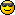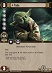Discussion about math, puzzles, games and fun.   Useful symbols: ÷ × ½ √ ∞ ≠ ≤ ≥ ≈ ⇒ ± ∈ Δ θ ∴ ∑ ∫  π  -¹ ² ³ °

You are not logged in.

## #1 2010-12-20 17:49:03

Mariner
Member
Registered: 2010-06-16
Posts: 35

### Finding a percentage of a number

I'm brushing up on my 'simple' maths before I go a do a placement test and I know for a fact that there are a number of questions that ask for a percentage of a number or quantity of something. I was wondering if I can have some tips on how to do these types of questions in my head or any 'tricks of the trade' if any.

The structure and style of questions asked are as below.
Find 25% of 5 hours.

Find 45% of 70 meters.

Find 5% of \$5

Thanks for the help:D

Offline

## #2 2010-12-20 17:59:50

bobbym
bumpkinFrom: Bumpkinland
Registered: 2009-04-12
Posts: 109,606

### Re: Finding a percentage of a number

H Mariner;

Welcome to the forum.

Find 5% of \$5

I do this type like this, move the decimal 1 place to the left:

Then divide by 2.

In mathematics, you don't understand things. You just get used to them.
If it ain't broke, fix it until it is.
Always satisfy the Prime Directive of getting the right answer above all else.

Offline

## #3 2010-12-21 13:33:53

stapel
Member
Registered: 2006-07-22
Posts: 15

### Re: Finding a percentage of a number

Mariner wrote:

...I know for a fact that there are a number of questions that ask for a percentage of a number or quantity of something. I was wondering if I can have some tips on how to do these types of questions in my head or any 'tricks of the trade' if any.

The general equation for is as follows:

. . . . .(this) is (some percent) of (that)

...where "is" means "equals" and "of" means "times".

You'll always be given two pieces of information. Plug those in, and solve for whatever is left, which will be what they're wanting.Last edited by bobbym (2013-03-18 01:20:20)

Offline

## #4 2010-12-28 04:07:02

John E. Franklin
MemberRegistered: 2005-08-29
Posts: 3,588

### Re: Finding a percentage of a number

I'll do 45% of 70.
double 45 to 90 while at the same time you half 70 to 35.
So now the problem is:
90% of 35.
This is easier. But how?
Remember that 90 is (100 - 10), so do this:
35 - 3.5 = answer
Right?
So 31.5.
Otherwise you could do it out in your head:
45% of 70.
.45
x7
2.80 + .35 = 3.15 but now I did 7 not 70, so 3.15 becomes 31.5 answer.

igloo myrtilles fourmis

Offline

## #5 2010-12-28 04:08:29

John E. Franklin
MemberRegistered: 2005-08-29
Posts: 3,588

### Re: Finding a percentage of a number

Now 25% of 5 hours is easy.
For 25% always half twice in a row.
half of 5 is 2.5
half of 2.5 is 1.25

igloo myrtilles fourmis

Offline

## #6 2010-12-28 04:10:06

John E. Franklin
MemberRegistered: 2005-08-29
Posts: 3,588

### Re: Finding a percentage of a number

If someone wants 20% of 120.
Then remember the 20% rule (divide by 5 rule).
Double and divide by ten (this works because 2/10 = 1/5)
So double 120 is 240 and then 240/10 is 24.

igloo myrtilles fourmis

Offline

## #7 2010-12-28 04:14:52

John E. Franklin
MemberRegistered: 2005-08-29
Posts: 3,588

### Re: Finding a percentage of a number

Now 80% of 136.
For 80% double 3 times and move over decimal place (2^3/10)
double 136 = 272
double 272 = 544
double 544 = 1088
move dot over left = 108.8

Another way is to subtract off 20% from 100% to get 80% using the 20% rule:
double 136 = 272, move dot over left, get 27.2, and subtract: 136 - 27.2 = 108.8

igloo myrtilles fourmis

Offline

## #8 2010-12-28 04:17:02

John E. Franklin
MemberRegistered: 2005-08-29
Posts: 3,588

### Re: Finding a percentage of a number

Now 75% of 144.
For 75%, think 3/4 because dividing by 4 is easy, just half twice.
Half of 144 is 72.
half of 72 is 36.
3 times 36 is 108 because (3 x 33) = 99 and 3 x 3 = 9 and 99 + 9 = 108.
(36 = 33 + 3)

igloo myrtilles fourmis

Offline

## #9 2010-12-28 04:18:59

John E. Franklin
MemberRegistered: 2005-08-29
Posts: 3,588

### Re: Finding a percentage of a number

Another one to know is 67 times 300% because it is so close to 66.6666666%
67 + 67 = 134
134 + 67 = 190 + 11 = 201
So 33.33333% of 201 is 67 also.
Note that 2 + 0 + 1 = 3, the 3 rule for division.

igloo myrtilles fourmis

Offline

## #10 2010-12-28 04:21:37

John E. Franklin
MemberRegistered: 2005-08-29
Posts: 3,588

### Re: Finding a percentage of a number

Also research The Trachtenberg Speed System of BASIC mathematics, though the techniques take time to learn.

igloo myrtilles fourmis

Offline

## #11 2016-08-17 15:44:30

Monox D. I-Fly
MemberFrom: Indonesia
Registered: 2015-12-02
Posts: 1,388

### Re: Finding a percentage of a number

Speaking of Trachtenberg system, does it have any method for speed subtraction?

Actually I never watch Star Wars and not interested in it anyway, but I choose a Yoda card as my avatar in honor of our great friend bobbym who has passed away. May his adventurous soul rest in peace at heaven.

Offline